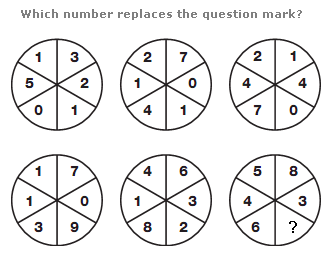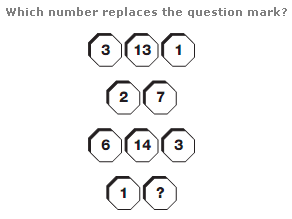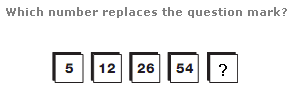# Puzzles - Number puzzles

### Exercise :: Number puzzlesAnswer : 1 Explanation : Starting with the circle on the top left, and moving to the right, top row then bottom row, the sum of the numbers in each circle follows the sequence 12, 15, 18, 21 etc.Answer : 4 Explanation : Split the whole diagram in half, horizontally, to give two groups of 5 octagons. In each group, the central number in the top row equals the sum of the surrounding 4 numbers.Answer : 110 Explanation : Starting on the left and moving right, double each number and add 2 to give the next number along.